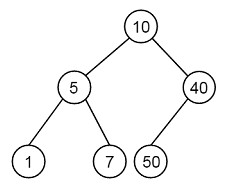# Construct BST from given preorder traversal - Set 2 in C++

Suppose we have one pre order traversal. From this traversal. we have to generate the tree So if the traversal is like [10, 5, 1, 7, 40, 50], then the tree will be like −To solve this, we will follow these steps −

• Create empty stack

• Make the first value as root, and push it into stack.

• Now keep pooping while the stack is not empty and the next value is greater than stack top element, make this as right child of the last popped node. Now push the new node to the stack.

• When the next value is less than the top element, make it as left child of stack top element. Now push the new node into the stack.

• Repeat the steps 2 and 3 until we have checked all of the preorder list elements.

## Example

Live Demo

#include <iostream>
#include <stack>
using namespace std;
class node {
public:
int data;
node *left;
node *right;
};
node* getNode (int data) {
node* temp = new node();
temp->data = data;
temp->left = temp->right = NULL;
return temp;
}
node* constructTree ( int pre[], int size ) {
stack<node*> stk;
node* root = getNode( pre );
stk.push(root);
int i;
node* temp;
for ( i = 1; i < size; ++i ) {
temp = NULL;
while ( !stk.empty() && pre[i] > stk.top()->data ) {
temp = stk.top();
stk.pop();
}
if ( temp != NULL) {
temp->right = getNode( pre[i] );
stk.push(temp->right);
} else {
node* peek_node = stk.top();
peek_node->left = getNode( pre[i] );
stk.push(stk.top()->left);
}
}
return root;
}
void inord (node* node) {
if (node == NULL)
return;
inord(node->left);
cout << node->data << " ";
inord(node->right);
}
int main () {
int pre[] = {10, 5, 1, 7, 40, 50};
int size = sizeof( pre ) / sizeof( pre );
node *root = constructTree(pre, size);
cout << "Inorder traversal: ";
inord(root);
}

### Output

Inorder traversal: 1 5 7 10 40 50